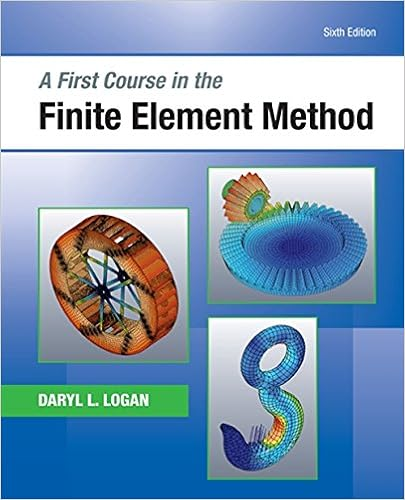# exam2_17_soln (1).pdf - ECH 141Fluid Mechanics Winter 2017...

• Test Prep
• 6
• 80% (5) 4 out of 5 people found this document helpful

This preview shows page 1 - 3 out of 6 pages.

##### We have textbook solutions for you!
The document you are viewing contains questions related to this textbook.The document you are viewing contains questions related to this textbook.
Chapter 14 / Exercise 14.2
A First Course in the Finite Element Method
LoganExpert Verified
ECH 141—Fluid Mechanics Winter 2017 Exam 2 2/1/2017 (One hour, Closed book, no notes) 1. In a coating process, a sheet of paper is pulled through a narrow slot filled with a coating fluid. the entrance to the slot (x=0) the dynamic pressure is P1, at the exit (x=L) the coating is exposed to air at and is at dynamic pressure P0, and the distance between the paper surfaces and the coating surfaces is H, as shown in the diagram below. The paper moves horizontally in the x-direction with velocity U, and the pressures at the entrance and exit are not equal. It may be assumed that L >> H. a) (20 pts.) Derive an expression for the velocity profile vX(y) in the region 0 y H. Assume that the pressures P1and P0are known. b) (10 pts.) Derive an expression for the flow rate Q of coating fluid through the slot, per unit width in the z-direction. c) (10 pts.) Derive an expression for the force FX, per unit width in the z-direction, needed to pull the paper sheet through the slot at velocity U. At Solutiona) Assume unidirectional, fully-developed, steady flow, then vx=vxy( )and vy=vz=0. The y-component of the Navier-Stokes equations shows that Py=0 or P=P x( )onlyso that the x-component simplifies to μd2vxdy2=dPdx=P1P0()L=ΔPL. Integrating twice yields vxy( )=12μΔPLy2+C1y+C2.
##### We have textbook solutions for you!
The document you are viewing contains questions related to this textbook.The document you are viewing contains questions related to this textbook.
Chapter 14 / Exercise 14.2
A First Course in the Finite Element Method
LoganExpert Verified
The boundary conditions are vx=0 at y=0and vx=U at y=HThe no-slip condition at y=0 yields C2=0. At y=H, U=12μΔPLH2+C1Hso C1=UH+12μΔPLHso vxy( )=12μΔPLHyy2+UHy. b) The flow rate per unit width in the z-direction is Q=vxy( )0Hdy=12μΔPLHyy2+UHy0Hdy. Then Q=12μΔPLH32H33+UH2or Q=H312μΔPL+UH2. c) The x-component of the force on a surface with a normal vector in the y-direction is calculated using the yx component of the shear stress, so for the force per unit width we need Fx=2τyxy=Hdx0L
.
•••